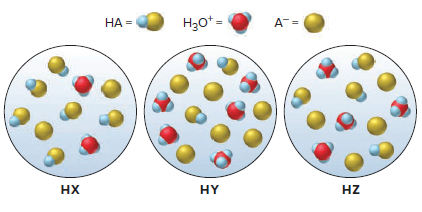# Problem: The following scene represent three weak acid HA (where A = X, Y, or Z) dissolved in water (H2O is not shown):(a) Rank the acids in order of increasing K a.

⚠️Our tutors found the solution shown to be helpful for the problem you're searching for. We don't have the exact solution yet.

###### Problem Details

The following scene represent three weak acid HA (where A = X, Y, or Z) dissolved in water (H2O is not shown):

(a) Rank the acids in order of increasing K a.